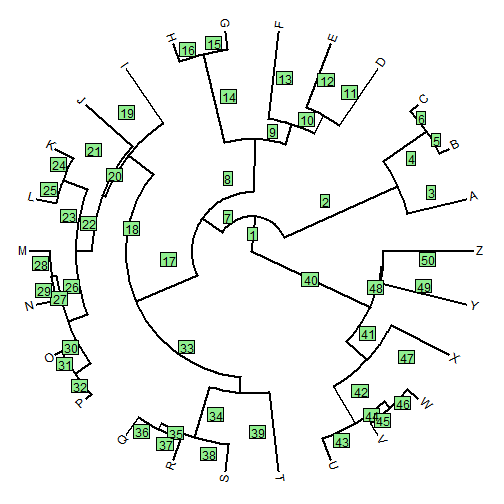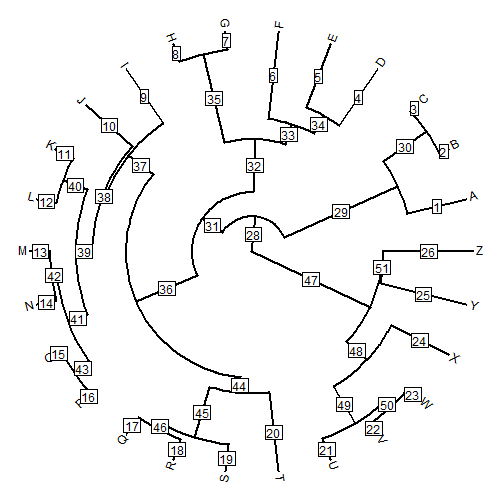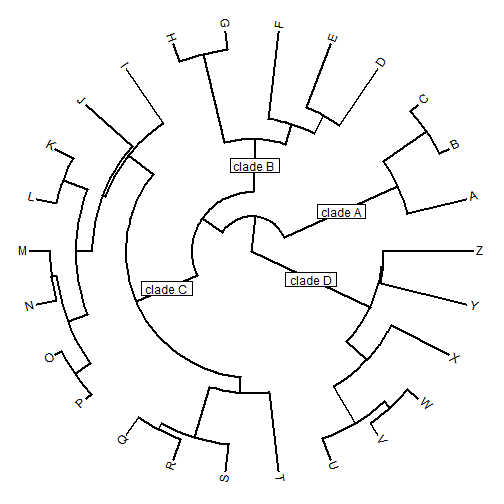## Thursday, July 30, 2015

### Edge labels on a radial or fan tree

Today on R-sig-phylo a reader reported a bug in the way that edge labels are plotted using the ape function `edgelabels`.

This error is very easy to reproduce:

``````set.seed(8) ## for repeatability
library(phytools)
tree<-pbtree(n=26,tip.label=LETTERS)
plotTree(tree,type="fan")
``````
``````## setEnv=TRUE for this type is experimental. please be patient with bugs
``````
``````edgelabels()
``````The problem is fairly clear to see. Instead of plotting the edge labels mid-way along each edge, the function is plotting them midway between the daughter node of the edge - and the daughter node of the parent edge. This does not work (except for edges descended directly from the root node).

[Another peculiarity of the function is that the plotted numbers are, by default, the rows of `phy\$edge` - not, for instance, the index of the daughter node of each edge. Let's ignore that for now.]

Luckily, Klaus Schliep, a postdoc presently working in my lab & author of the phangorn package quickly leapt to the rescue with a complete bug fix. However, a little too late I also had written a little code which, though not as complete as Klaus's fix, nonetheless might be instructive (with comments below) on how these functions work.

Here is my function, which by default plots numbers corresponding to the daughter of each edge - not the row of `phy\$edge`:

``````edgelabels.fan<-function(text=NULL,edge=NULL,cex=1){
## get the last plotted "phylo" object - created by
## plot.phylo, plotTree, and other methods
obj<-get("last_plot.phylo",envir=.PlotPhyloEnv)
## these are the positions of the nodes & tips in the plotted
## tree
nx<-obj\$xx[obj\$edge[,2]]
ny<-obj\$yy[obj\$edge[,2]]
## this function computes the length of each edge from the
## object we pulled, above
foo<-function(e,x,y) sqrt(x[e]^2+y[e]^2)-
sqrt(x[e]^2+y[e]^2)
l<-apply(obj\$edge,1,foo,x=obj\$xx,y=obj\$yy)
## now let's get the x & y coordinates of the midpoint
## of each edge
x<-nx-sign(nx)*0.5*l*cos(atan(ny/nx))
y<-ny-sign(nx)*0.5*l*sin(atan(ny/nx))
## some bookkeeping
ii<-order(as.numeric(names(x)))
x<-x[ii]
y<-y[ii]
if(is.null(edge)) edge<-c(1:obj\$Ntip,2:obj\$Nnode+obj\$Ntip)
x<-x[as.character(edge)]
y<-y[as.character(edge)]
if(is.null(text)) text<-names(x)
if(length(text)!=length(edge)){
cat("Warning: edge & text should be the same length.\n\n")
text<-as.character(edge)
}
## let's plot our edge labels
rectangles=cbind(1.2*cex*strwidth(text),
1.4*cex*strheight(text)))
text(text,x=x,y=y,cex=cex)
}
``````

OK, now we might as well try it out:

``````plotTree(tree,type="fan")
``````
``````## setEnv=TRUE for this type is experimental. please be patient with bugs
``````
``````edgelabels.fan()
``````or, alternatively:

``````plotTree(tree,type="fan")
``````
``````## setEnv=TRUE for this type is experimental. please be patient with bugs
``````
``````edgelabels.fan(edge=c(29,32,36,47),